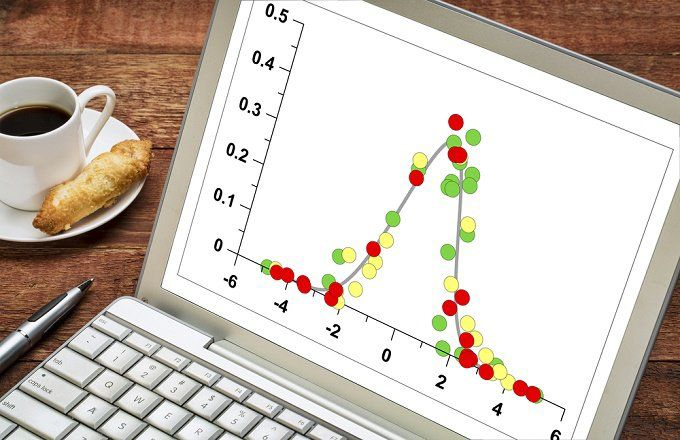Press "Enter" to skip to content

The mathematics behind finance can be a bit confusing and tedious. Luckily, most computer programs do complex calculations. However, understanding the various statistical terms and methods, their meanings, and which best analyzes investments is crucial when picking the appropriate security and getting the desired impact on a portfolio.

One important decision is choosing between normal versus lognormal distributions, both are often referred to in research literature. Before choosing, you need to know:

• What they are
• What differences exist between them
• How they impact investment decisions

### Normal Versus Lognormal

Both normal and lognormal distributions are used in statistical mathematics to describe the probability of an event occurring. Flipping a coin is an easily understood example of probability. If you flip a coin 1000 times, what is the distribution of results? That is, how many times will it land on heads or tails? There is a 50% probability that it will land on either heads or tails. This basic example describes the probability and distribution of results. (For details, see “Basics of the Binomial Distribution.”)

There are many types of distributions, one of which is the normal or bell curve distribution. (See figure 1.)

In a normal distribution, 68% (34%+34%) of the results fall within one standard deviation, and 95% (68%+13.5%+13.5%) fall within two standard deviations. At the center (the 0 point in the image above) the median (the middle value in the set), the mode (the value that occurs most often), and the mean (arithmetic average) are all the same.

The lognormal distribution differs from the normal distribution in several ways. A major difference is in its shape: the normal distribution is symmetrical, whereas the lognormal distribution is not. Because the values in a lognormal distribution are positive, they create a right-skewed curve. (See Fig 2)

This skewness is important in determining which distribution is appropriate to use in investment decision-making. A further distinction is that the values used to derive a lognormal distribution are normally distributed.

Let’s clarify with an example. An investor wants to know an expected future stock price. Since stocks grow at a compounded rate, she needs to use a growth factor. To calculate possible expected prices, she will take the current stock price and multiply it by various rates of return (which are mathematically derived exponential factors based on compounding), which are assumed to be normally distributed. When the investor continuously compounds the returns, she creates a lognormal distribution. This distribution is always positive even if some of the rates of return are negative, which will happen 50% of the time in a normal distribution. The future stock price will always be positive because stock prices cannot fall below \$0.

### When to Use Normal Versus Lognormal Distribution

The preceding example helped us arrive at what really matters to investors: when to use each method. Lognormal is extremely useful when analyzing stock prices. As long as the growth factor used is assumed to be normally distributed (as we assume with the rate of return), then the lognormal distribution makes sense. Normal distribution cannot be used to model stock prices because it has a negative side, and stock prices cannot fall below zero.

Conversely, normal distribution works better when calculating total portfolio returns. The normal distribution is used because the weighted average return (the product of the weight of a security in a portfolio and its rate of return) is more accurate in describing the actual portfolio return (positive or negative), particularly if the weights vary by a large degree. The following is a typical example:

Portfolio Holdings           Weights             Returns              Weighted Return

Stock A                                40%                       12%                      40% * 12% = 4.8%

Stock B                                60%                       6%                         60% * 6% = 3.6%

Total Weighted Average Return = 4.8% + 3.6% = 8.4%

Although the lognormal return for total portfolio performance may be quicker to calculate over a longer time period, it fails to capture the individual stock weights, which can distort the return tremendously. Also, portfolio returns can be positive or negative, and a lognormal distribution will fail to capture the negative aspects.

### The Bottom Line

Although the nuances that differentiate normal and lognormal distributions may escape us most of the time, knowledge of the appearance and characteristics of each distribution will provide insight into how to model portfolio returns and future stock prices.https://www.investopedia.com/articles/investing/102014/lognormal-and-normal-distribution.asp?utm_campaign=rss_articles&utm_source=rss&utm_medium=referral